# Search by Topic

#### Resources tagged with Volume and capacity similar to 2D-3D:

Filter by: Content type:
Age range:
Challenge level:

### There are 16 results

Broad Topics > Measuring and calculating with units > Volume and capacity### 2D-3D

##### Age 16 to 18 Challenge Level:

Two circles of equal size intersect and the centre of each circle is on the circumference of the other. What is the area of the intersection? Now imagine that the diagram represents two spheres of. . . .### Reach for Polydron

##### Age 16 to 18 Challenge Level:

A tetrahedron has two identical equilateral triangles faces, of side length 1 unit. The other two faces are right angled isosceles triangles. Find the exact volume of the tetrahedron.### In a Spin

##### Age 14 to 16 Challenge Level:

What is the volume of the solid formed by rotating this right angled triangle about the hypotenuse?### Tubular Stand

##### Age 14 to 16 Challenge Level:

If the radius of the tubing used to make this stand is r cm, what is the volume of tubing used?
### Concrete Calculation

##### Age 14 to 16 Challenge Level:

The builders have dug a hole in the ground to be filled with concrete for the foundations of our garage. How many cubic metres of ready-mix concrete should the builders order to fill this hole to. . . .### Three Cubes

##### Age 14 to 16 Challenge Level:

Can you work out the dimensions of the three cubes?### Immersion

##### Age 14 to 16 Challenge Level:

Various solids are lowered into a beaker of water. How does the water level rise in each case?### Maths Filler 2

##### Age 14 to 16 Challenge Level:

Can you draw the height-time chart as this complicated vessel fills with water?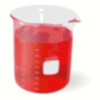### Brimful 2

##### Age 16 to 18 Challenge Level:

Which of these infinitely deep vessels will eventually full up?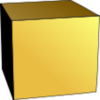### Uniform Units

##### Age 14 to 16 Challenge Level:

Can you choose your units so that a cube has the same numerical value for it volume, surface area and total edge length?### Plane to See

##### Age 16 to 18 Challenge Level:

P is the midpoint of an edge of a cube and Q divides another edge in the ratio 1 to 4. Find the ratio of the volumes of the two pieces of the cube cut by a plane through PQ and a vertex.### Mouhefanggai

##### Age 14 to 16

Imagine two identical cylindrical pipes meeting at right angles and think about the shape of the space which belongs to both pipes. Early Chinese mathematicians call this shape the mouhefanggai.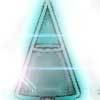### Conical Bottle

##### Age 14 to 16 Challenge Level:

A right circular cone is filled with liquid to a depth of half its vertical height. The cone is inverted. How high up the vertical height of the cone will the liquid rise?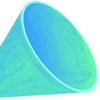### Funnel

##### Age 14 to 16 Challenge Level:

A plastic funnel is used to pour liquids through narrow apertures. What shape funnel would use the least amount of plastic to manufacture for any specific volume ?### Sliced

##### Age 14 to 16 Challenge Level:

An irregular tetrahedron has two opposite sides the same length a and the line joining their midpoints is perpendicular to these two edges and is of length b. What is the volume of the tetrahedron?### Cuboid Challenge

##### Age 11 to 16 Challenge Level:

What's the largest volume of box you can make from a square of paper?